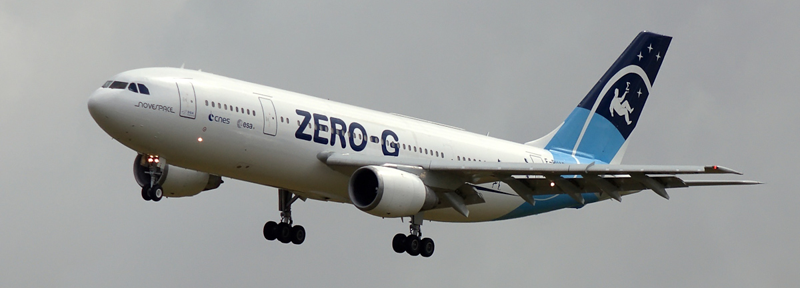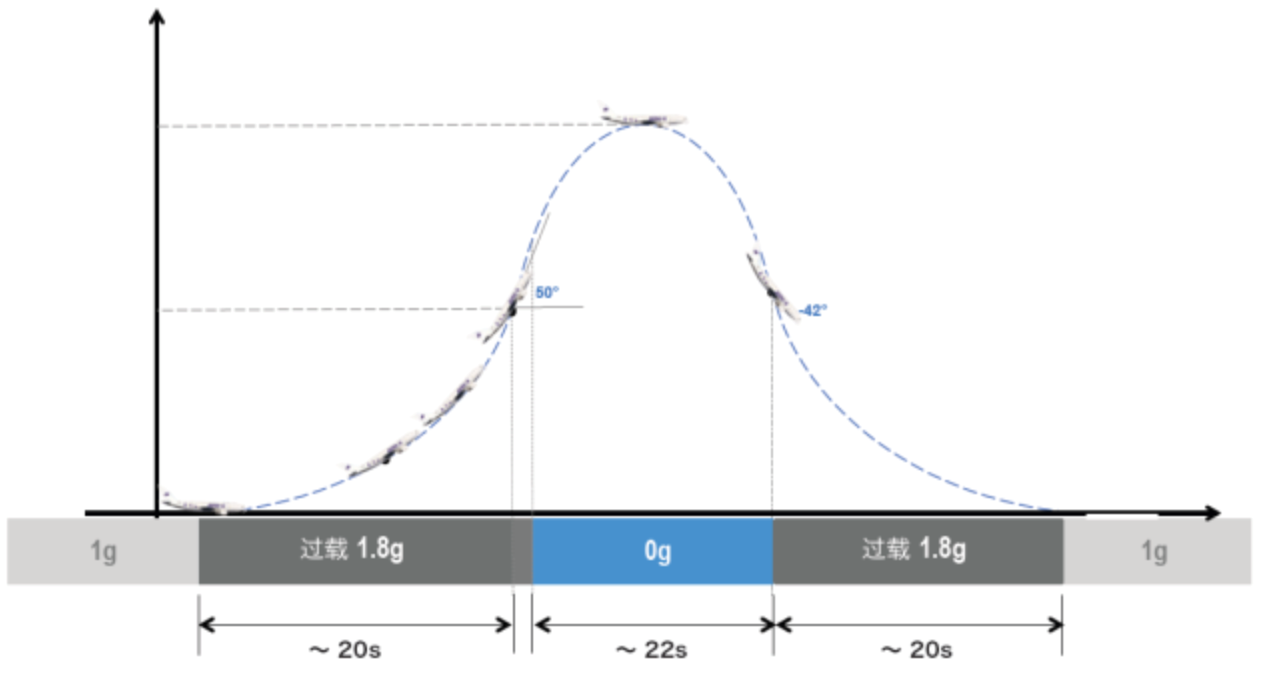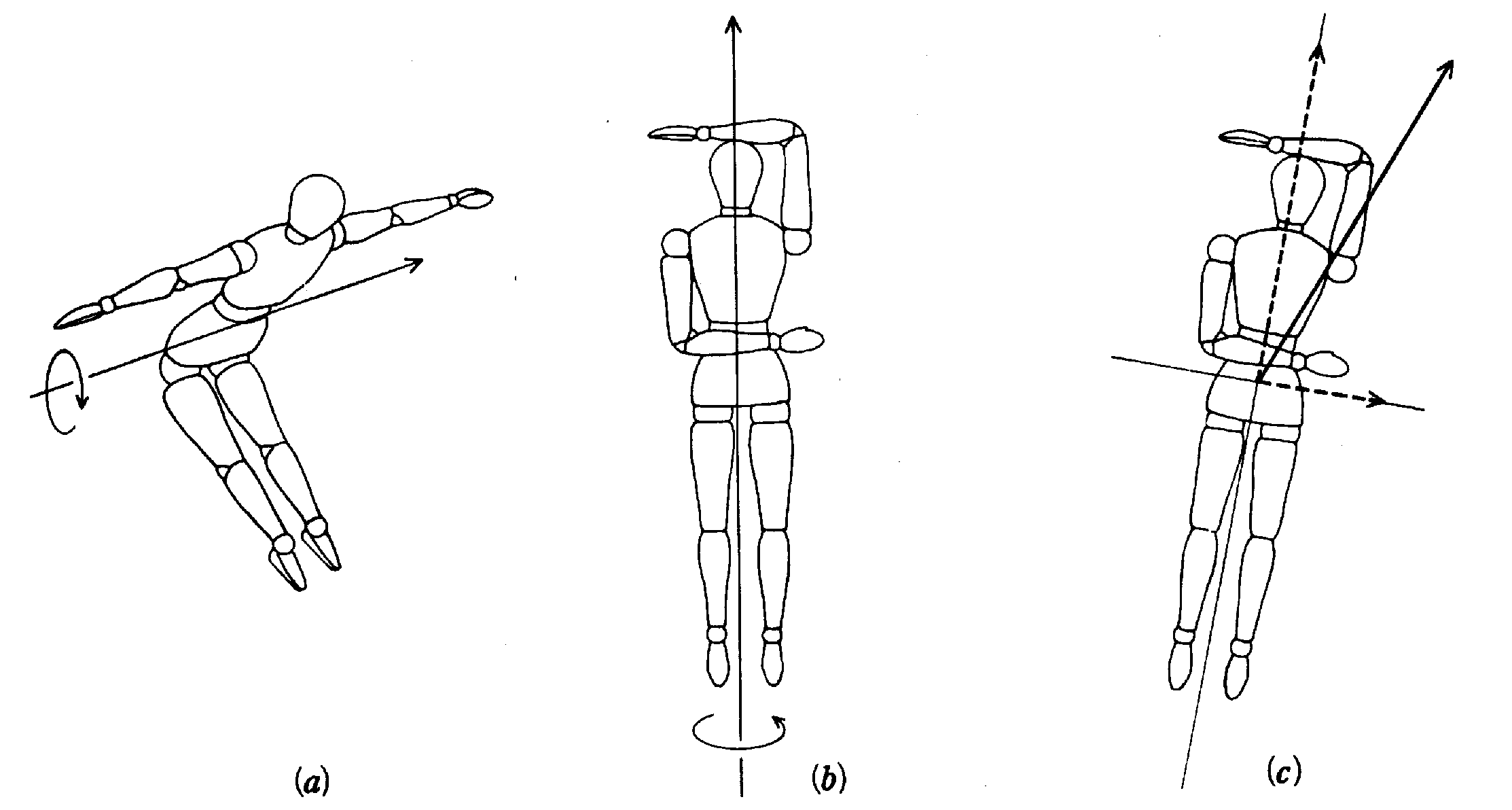# 失重飞机笔记: 挑战吧太空观察记录

2 分钟阅读

## 失重和超重## Novespace Zero-G 的飞行轨迹$\Delta h_0 = \frac 12 a t_0^2 = 1568 \mathrm{m}$

$v_\perp = a t = 157 \mathrm{m/s}$

$v_\parallel = v_\perp / \tan 50° = 132\mathrm{m/s}$

$v_\perp' = -v_\parallel \tan 42° = -118\mathrm{m/s}$

$\Delta h' = v_\perp^2/(2 a) = 1254 \mathrm{m}$## 人在失重条件下的姿态控制和转动惯量